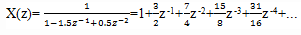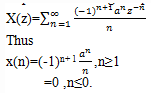Test: Z Transform Inversion

# Test: Z Transform Inversion

Test Description

## 10 Questions MCQ Test Digital Signal Processing | Test: Z Transform Inversion

Test: Z Transform Inversion for Electrical Engineering (EE) 2022 is part of Digital Signal Processing preparation. The Test: Z Transform Inversion questions and answers have been prepared according to the Electrical Engineering (EE) exam syllabus.The Test: Z Transform Inversion MCQs are made for Electrical Engineering (EE) 2022 Exam. Find important definitions, questions, notes, meanings, examples, exercises, MCQs and online tests for Test: Z Transform Inversion below.
Solutions of Test: Z Transform Inversion questions in English are available as part of our Digital Signal Processing for Electrical Engineering (EE) & Test: Z Transform Inversion solutions in Hindi for Digital Signal Processing course. Download more important topics, notes, lectures and mock test series for Electrical Engineering (EE) Exam by signing up for free. Attempt Test: Z Transform Inversion | 10 questions in 10 minutes | Mock test for Electrical Engineering (EE) preparation | Free important questions MCQ to study Digital Signal Processing for Electrical Engineering (EE) Exam | Download free PDF with solutions
 1 Crore+ students have signed up on EduRev. Have you?
Test: Z Transform Inversion - Question 1

### Which of the following method is used to find the inverse z-transform of a signal?

Detailed Solution for Test: Z Transform Inversion - Question 1

Explanation: All the methods mentioned above can be used to calculate the inverse z-transform of the given signal.

Test: Z Transform Inversion - Question 2

### What is the inverse z-transform of X(z)=1/(1-1.5z-1+0.5z-2 ) if ROC is |z|>1?

Detailed Solution for Test: Z Transform Inversion - Question 2

Explanation: Since the ROC is the exterior circle, we expect x(n) to be a causal signal. Thus we seek a power series expansion in negative powers of ‘z’. By dividing the numerator of X(z) by its denominator, we obtain the power seriesSo, we obtain x(n)= {1,3/2,7/4,15/8,31/16,….}.

Test: Z Transform Inversion - Question 3

### What is the inverse z-transform of X(z)=log(1+az-1) |z|>|a|?

Detailed Solution for Test: Z Transform Inversion - Question 3

Explanation: Using the power series expansion for log(1+x), with |x|<1, we haveTest: Z Transform Inversion - Question 4

What is the proper fraction and polynomial form of the improper rational transform
X(z)= (1+3z-1+11/6 z-2+1/3 z-3)/(1+5/6 z-1+1/6 z-2 )?

Detailed Solution for Test: Z Transform Inversion - Question 4

Explanation: First, we note that we should reduce the numerator so that the terms z-2 and z -3 are eliminated. Thus we should carry out the long division with these two polynomials written in the reverse order. We stop the division when the order of the remainder becomes z -1. Then we obtain
X(z)= 1+2z -1+(1/6 z-1)/(1+5/6 z-1+1/6 z-2 ).

Test: Z Transform Inversion - Question 5

What is the partial fraction expansion of the proper function X(z)= 1/(1-1.5z-1+0.5z-2 )?

Detailed Solution for Test: Z Transform Inversion - Question 5

Explanation: First we eliminate the negative powers of z by multiplying both numerator and denominator by z2.
Thus we obtain X(z)= z2/(z2-1.5z+0.5)
The poles of X(z) are p1=1 and p2=0.5. Consequently, the expansion will be
(X(z))/z = z/((z-1)(z-0.5)) = 2/((z-1) ) – 1/((z-0.5) )( obtained by applying partial fractions)
=>X(z)= 2z/(z-1)-z/(z-0.5).

Test: Z Transform Inversion - Question 6

What is the partial fraction expansion of X(z)= (1+z-1)/(1-z-1+0.5z-2 )?

Detailed Solution for Test: Z Transform Inversion - Question 6

Explanation: To eliminate the negative powers of z, we multiply both numerator and denominator by z2. Thus,
X(z)=(z(z+1))/(z-2-z+0.5)
The poles of X(z) are complex conjugates p1=0.5+0.5j and p2=0.5-0.5j
Consequently the expansion will be
X(z)= (z(0.5-1.5j))/(z-0.5-0.5j) + (z(0.5+1.5j))/(z-0.5+0.5j).

Test: Z Transform Inversion - Question 7

What is the partial fraction expansion of X(z)=1/((1+z-1 )(1-z-1)2)?

Detailed Solution for Test: Z Transform Inversion - Question 7

Explanation: First we express X(z) in terms of positive powers of z, in the form X(z)=z3/((z+1)〖(z-1)〗2 )
X(z) has a simple pole at z=-1 and a double pole at z=1. In such a case the approximate partial fraction expansion is
(X(z))/z = z2/((z+1)〖(z-1)〗2 ) =A/(z+1) + B/(z-1) + C/〖(z-1)〗2
On simplifying, we get the values of A, B and C as 1/4, 3/4 and 1/2 respectively.
Therefore, we get X(z)= z/(4(z+1)) + 3z/(4(z-1)) + z/(2〖(z-1)〗2 ) .

Test: Z Transform Inversion - Question 8

What is the inverse z-transform of X(z)= 1/(1-1.5z-1+0.5z2-2 ) if ROC is |z|>1?

Detailed Solution for Test: Z Transform Inversion - Question 8

Explanation: The partial fraction expansion for the given X(z) is
X(z)= 2z/(z-1)-z/(z-0.5)
In case when ROC is |z|>1, the signal x(n) is causal and both the terms in the above equation are causal terms. Thus, when we apply inverse z-transform to the above equation, we get
x(n)=2(1)nu(n)-(0.5)nu(n)=(2-0.5n)u(n).

Test: Z Transform Inversion - Question 9

What is the inverse z-transform of X(z)= 1/(1-1.5z-1+0.5z-2 ) if ROC is |z|<0.5?

Detailed Solution for Test: Z Transform Inversion - Question 9

Explanation: The partial fraction expansion for the given X(z) is
X(z)= 2z/(z-1)-z/(z-0.5)
In case when ROC is |z|<0.5,the signal is anti causal. Thus both the terms in the above equation are anti causal terms. So, if we apply inverse z-transform to the above equation we get x(n)= [-2+0.5n]u(-n-1).

Test: Z Transform Inversion - Question 10

What is the inverse z-transform of X(z)= 1/(1-1.5z-1+0.5z-2 ) if ROC is 0.5<|z|<1?

Detailed Solution for Test: Z Transform Inversion - Question 10

Explanation: The partial fraction expansion of the given X(z) is
X(z)= 2z/(z-1)-z/(z-0.5)
In this case ROC is 0.5<|z|<1 is a ring, which implies that the signal is two sided. Thus one of the signal corresponds to a causal signal and the other corresponds to an anti causal signal. Obviously, the ROC given is the overlapping of the regions |z|>0.5 and |z|<1. Hence the pole p2=0.5 provides the causal part and the pole p1=1 provides the anti causal part. SO, if we apply the inverse z-transform we get x(n)= -2u(-n-1)-(0.5)nu(n).

## Digital Signal Processing

3 videos|50 docs|54 tests
 Use Code STAYHOME200 and get INR 200 additional OFF Use Coupon Code
Information about Test: Z Transform Inversion Page
In this test you can find the Exam questions for Test: Z Transform Inversion solved & explained in the simplest way possible. Besides giving Questions and answers for Test: Z Transform Inversion, EduRev gives you an ample number of Online tests for practice

## Digital Signal Processing

3 videos|50 docs|54 tests